# phased.LinearFMWaveform

Linear FM pulse waveform

## Description

The LinearFMWaveform System object™ creates a linear FM pulse waveform.

To create the waveform:

1. Create the phased.LinearFMWaveform object and set its properties.

2. Call the object with arguments, as if it were a function.

## Creation

### Syntax

waveform = phased.LinearFMWaveform
waveform = phased.LinearFMWaveform(Name=Value)

### Description

example

waveform = phased.LinearFMWaveform creates a linear FM pulse waveform System object. Use this object to generate samples of a linear FM pulse waveform.
waveform = phased.LinearFMWaveform(Name=Value) creates a linear FM pulse waveform System object with each specified property Name set to the specified Value. You can specify additional name-value pair arguments in any order as (Name1=Value1,...,NameN=ValueN).

## Properties

expand all

Unless otherwise indicated, properties are nontunable, which means you cannot change their values after calling the object. Objects lock when you call them, and the release function unlocks them.

If a property is tunable, you can change its value at any time.

Signal sample rate, specified as a positive scalar. The ratio of sample rate to pulse repetition frequency must be a positive integer, so the number of samples in each pulse must be an integer value. Units are in Hertz.

Example: 100e3

Data Types: double

Method to set pulse duration (pulse width), specified as 'Pulse width' or 'Duty cycle'. This property determines how you set the pulse duration.

• When you set this property to 'Pulse width', set the pulse duration directly using the PulseWidth property.

• When you set this property to 'Duty cycle', set the pulse duration from the values of the PRF and DutyCycle properties. The pulse width is equal to the value of the DutyCycle property divided by the value of the PRF property.

Data Types: char | string

Pulse time duration, specified as a positive scalar. The value must satisfy PulseWidth <= 1./PRF. Units are in seconds.

#### Dependencies

To enable this property, set the DurationSpecification property to 'Pulse width'.

Data Types: double

Pulse duty cycle, specified as a positive scalar in the range [0,1]. The pulse width is the value of the DutyCycle property divided by the value of the PRF property. This quantity is dimensionless.

Example: 0.75

#### Dependencies

To enable this property, set the DurationSpecification property to 'Duty cycle'.

Data Types: double

Pulse repetition frequency (PRF), specified as a scalar or a row vector. Units are in Hz. The pulse repetition interval (PRI) is the inverse of the pulse repetition frequency PRF value. The PRF must satisfy these restrictions:

• The product of PRF and PulseWidth must be less than or equal to one. This condition requires that the pulse width is less than one PRI. For the phase-coded waveform, the pulse width is the product of the values of the ChipWidth and NumChips properties.

• The ratio of SampleRate to PRF must be an integer. This condition requires that the number of samples in one PRI is an integer.

You can set the value of PRF using the PRF property settings alone or using property settings in conjunction with the prfidx input argument of the object.

• When PRFSelectionInputPort is false, you set the PRF using the PRF properties alone. You can:

• Implement a constant PRF by specifying the PRF property as a positive real-valued scalar.

• Implement a staggered PRF by specifying the PRF property as a row vector with positive real-valued elements. Each call to the object uses successive elements of this vector as the PRF. Once the object reaches the last element of the vector, it continues the process cyclically with the first element of the vector.

• When PRFSelectionInputPort is true, you can set the PRF value using the PRF property in conjunction with the prfidx input argument. You implement a selectable PRF by specifying the PRF property as a row vector with positive real-valued elements. When you execute the object, the object selects a PRF by using the index you specify in the prfidx input argument to index into the PRF vector.

In all cases, the number of output samples is fixed when you set the OutputFormat property to 'Samples'. When you use a varying PRF and also set the OutputFormat property to 'Pulses', the number of samples can vary.

Data Types: double

Enable PRF selection input, specified as false or true. When you set this property to true, you can pass an index argument to the object to select a predefined value from the PRF property vector. When you set this property to false, the object uses the PRF property to define the PRF sequence used in the simulation.

Data Types: logical

Bandwidth of linear FM sweep, specified as a positive scalar. Units are in Hz.

Data Types: double

Direction of the linear FM sweep, specified as 'Up' or 'Down'.

Data Types: char | string

Location of the FM sweep interval, specified as'Positive' or 'Symmetric'.

• If SweepInterval is 'Positive', the waveform sweeps the interval between 0 and B where B is the sweep bandwidth in the SweepBandwidth property.

• If SweepInterval is 'Symmetric', the waveform sweeps the interval between -B/2 and B/2.

Data Types: char | string

Waveform envelope function, specified as 'Rectangular' or 'Gaussian'.

Data Types: char | string

Source of frequency offset, specified as 'Property' or 'Input port'.

• When you set this property to 'Property', the frequency offset is determined by the value of the FrequencyOffset property.

• When you set this property to 'Input port', the frequency offset is determined by the input argument freqoffset when calling the object.

Example: 'Input port'

Data Types: char | string

Frequency offset, specified as a scalar. Units are in Hz.

Example: 150.0

#### Dependencies

To enable this property, set the FrequencyOffsetSource property to 'Property'.

Data Types: double

Format of output signal, specified as 'Pulses' or 'Samples'.

• When you set the OutputFormat property to 'Pulses', the output of the object takes the form of multiple pulses specified by the value of the NumPulses property. The number of samples per pulse can vary if you change the PRF during the simulation.

• When you set the OutputFormat property to 'Samples', the output of the object takes the form of multiple samples. In this case, the number of output signal samples is the value of the NumSamples property and is fixed.

Data Types: char | string

Number of samples in each output of the object, specified as a positive integer.

#### Dependencies

To enable this property, set the OutputFormat property to 'Samples'.

Data Types: double

Number of pulses in each output, specified as a positive integer.

#### Dependencies

To enable this property, set the OutputFormat property to 'Pulses'.

Data Types: double

Enable PRF output, specified as false or true. Set this property to true to output the PRF.

#### Dependencies

To enable this property, set the OutputFormat property to 'Pulses'.

Data Types: logical

Enable matched filter coefficients output, specified as false or true. Set this property to true to enable the object the output of the matched filter coefficients of the waveform used during the simulation.

Data Types: logical

## Usage

### Syntax

Y = waveform()
Y = waveform(prfidx)
Y = waveform(freqoffset)
[Y,PRF] = waveform(___)
[Y,coeff] = waveform(___)

### Description

example

Y = waveform() returns samples of the linear FM pulse in a column vector Y. Y can contain either a certain number of pulses or a certain number of samples.
Y = waveform(prfidx), specifies the index prfidx of the pulse repetition frequency (PRF). The index identifies selected entries in the PRF property. This syntax applies when you set the PRFSelectionInputPort property to true.Use this syntax for the cases where the transmitted pulse needs to be dynamically selected. In such situations, the PRF property includes a list of predetermined choices of PRF's. Based on prfidx value input, one of the PRF's is selected as the PRF for the next transmission.Note that the transmission always finishes the current pulse before starting the next pulse. Therefore, when you set the OutputFormat property to 'Samples' and then specify the NumSamples property to be shorter than a pulse, it is possible that during a given simulation step, if the entire output is needed to finish the previously transmitted pulse, the specified prfidx is ignored.
Y = waveform(freqoffset) generates a waveform with a frequency offset freqoffset. Use this syntax for cases where the transmit pulse frequency needs to be dynamically updated.This syntax applies when you set the FrequencyOffsetSource property to 'Input port'.
[Y,PRF] = waveform(___) also returns the current pulse repetition frequency PRF. To enable this syntax, set the PRFOutputPort property to true and set the OutputFormat property to 'Pulses'.
[Y,coeff] = waveform(___) also returns the matched filter coefficients, coeff, for the current pulse. To enable this syntax, set the CoefficientsOutputPort property to true.

You can combine optional input and output arguments when their enabling properties are set. Optional inputs and outputs must be listed in the same order as the order of the enabling properties. For example,

[Y,PRF,coeff] = waveform(prfidx,freqoffset)

### Input Arguments

expand all

Index of pulse frequency, specified as a positive integer. The index identifies the entries in the PRF.

#### Dependencies

To enable this argument, set the PRFSelectionInputPort property to true

Frequency offset, specified as a scalar. The offset generates the waveform with a frequency offset. Use this argument for the cases where the transmit pulse frequency needs to be dynamically updated. Units are in Hz.

#### Dependencies

To enable this argument, set the FrequencyOffsetSource property to 'Input port'.

Data Types: double

### Output Arguments

expand all

Pulse repetition frequency, returned as a scalar.

#### Dependencies

To enable this argument, set the PRFOutputPort property to true and set the OutputFormat to 'Pulses'. When you set the PRFOutputPort property to true, the function returns the current PRF used by the system.

Matched filter coefficients, returned as an NZ-by-1 complex-valued vector or an NZ-by-M complex-valued matrix.

• If you set OutputFormat to 'Pulses' and NumPulses is 1, the object returns coeff as an NZ-length vector. NZ corresponds to the pulse width.

• If you set OutputFormat to 'Pulses' with NumPulses greater than 1 or OutputFormat is 'Samples' and DurationSpecification is 'Pulse width', coeff is returned as an NZ-length vector. NZ corresponds to the pulse width.

• If OutputFormat is set to 'Pulses' with NumPulses greater than 1 or OutputFormat is set to 'Samples' and DurationSpecification is set to 'Duty cycle' with only one unique PRF value, coeff is returned as an NZ-length vector. NZ corresponds to the pulse width.

• If OutputFormat is 'Pulses' with NumPulses greater than 1 or OutputFormat is 'Samples' and DurationSpecification is 'Duty cycle', coeff is returned as an NZ-by-M matrix. NZ corresponds to the maximum pulse width and M corresponds to the number of unique PRFs.

#### Dependencies

To enable this argument, set the CoefficientsOutputPort property to true.

Data Types: double
Complex Number Support: Yes

## Object Functions

To use an object function, specify the System object as the first input argument. For example, to release system resources of a System object named obj, use this syntax:

release(obj)

expand all

 bandwidth Waveform bandwidth getMatchedFilter Matched filter coefficients derived from waveform getStretchProcessor Create stretch processor for waveform plot Plot pulse waveform
 step Run System object algorithm release Release resources and allow changes to System object property values and input characteristics reset Reset internal states of System object

## Examples

collapse all

Construct a linear FM waveform having a sweep bandwidth of 300 kHz, a sample rate of 1 MHz, a pulse width of 50 microseconds, and a pulse repetition frequency of 10 kHz. Generate two pulses.

sLFM = phased.LinearFMWaveform('SweepBandwidth',3e5,... 'OutputFormat','Pulses','SampleRate',1e6,... 'PulseWidth',50e-6,'PRF',10e3,'NumPulses',2);

Obtain and plot the linear FM waveform.

wav = step(sLFM); numpulses = size(wav,1); t = [0:(numpulses-1)]/sLFM.SampleRate; plot(t*1e6,real(wav)) xlabel('Time (\mu sec)') ylabel('Amplitude')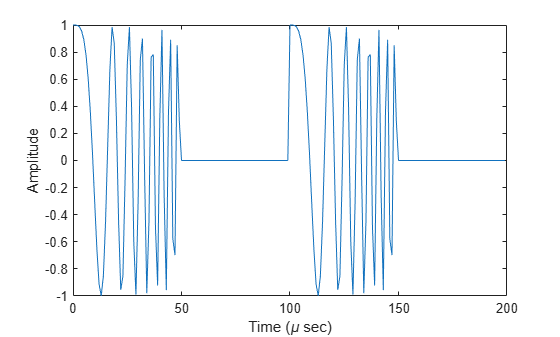Construct six linear FM waveform pulses having a sweep bandwidth of 300 kHz, a sample rate of 1 MHz, a pulse width of 50 microseconds, and a duty cycle of 20%. Vary the pulse repetition frequency.

Set the sample rate and PRF. The ratio of sample rate to PRF must be an integer.

fs = 1e6; PRF = [10000,25000]; sLFM = phased.LinearFMWaveform('SweepBandwidth',3e5,... 'OutputFormat','Pulses','SampleRate',fs,... 'DurationSpecification','Duty Cycle','DutyCycle',.2,... 'PRF',PRF,'NumPulses',1,'PRFSelectionInputPort',true);

Obtain and plot the linear FM waveforms. For the first three calls to the step method, set the PRF to 10 kHz using the PRF index. For the next three calls, set the PRF to 25 kHz.

wav = []; for n = 1:6 idx = floor((n-1)/3)+1; wav1 = step(sLFM,idx); wav = [wav;wav1]; end nsamps = size(wav,1); t = [0:(nsamps-1)]/sLFM.SampleRate; plot(t*1e6,real(wav)) xlabel('Time (\mu sec)') ylabel('Amplitude')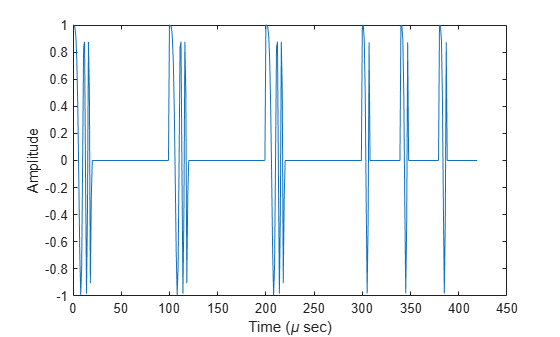Create and plot an upsweep linear FM pulse waveform. The sample rate is 500 kHz, the sweep bandwidth is 200 kHz and the pulse width is 1 millisecond (equal to the pulse repetition interval).

fs = 500e3; sLFM = phased.LinearFMWaveform('SampleRate',fs,... 'SweepBandwidth',200e3,... 'PulseWidth',1e-3,'PRF',1e3);

Obtain and then plot the real part of the LFM waveform.

lfmwav = step(sLFM); nsamp = size(lfmwav,1); t = [0:(nsamp-1)]/fs; plot(t*1000,real(lfmwav)) xlabel('Time (millisec)') ylabel('Amplitude') grid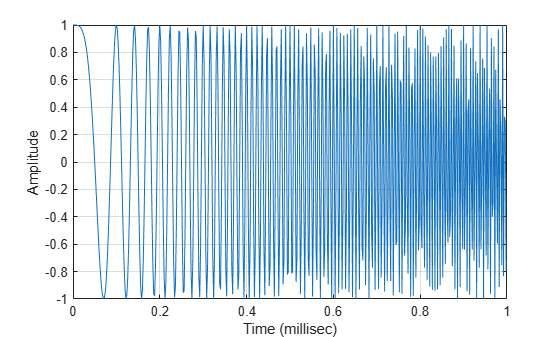Plot the Fourier transform of the complex signal.

nfft = 2^nextpow2(nsamp); Z = fft(lfmwav,nfft); fr = [0:(nfft/2-1)]/nfft*fs; plot(fr/1000,abs(Z(1:nfft/2)),'.-') xlabel('Frequency (kHz)') ylabel('Amplitude') grid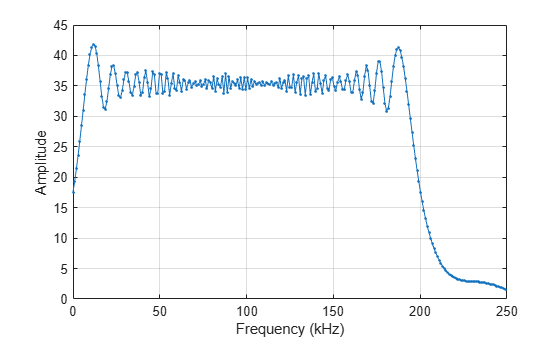Plot a spectrogram of the function with window size of 64 samples and 50% overlap.

nfft1 = 64; nov = floor(0.5*nfft1); spectrogram(lfmwav,hamming(nfft1),nov,nfft1,fs,'centered','yaxis')This plot shows the increasing frequency of the signal.

Apply a frequency offset to an upsweep linear FM (LFM) pulse waveform. Plot the frequency spectrum of the waveform with and without applying a frequency offset.

Create an LFM waveform object, which is configured to set the frequency offset from an input when the object is executed.

fs = 500e3; sLFM = phased.LinearFMWaveform('SampleRate',fs,'SweepBandwidth',200e3, ... 'PulseWidth',2e-5,'PRF',1e3,'FrequencyOffsetSource','Input port');

Execute the object two times. First set the frequency offset to 0 Hz and then to 2e4 Hz.

lfmwav = sLFM(0); lfmwav_foffset = sLFM(2e4);

Plot the frequency spectrum of the complex signals. The frequency offset signal is shifted to the right.

[Pxx,f] = pwelch(lfmwav,[],[],[],fs,'centered'); [Pxx_offset,foffset] = pwelch(lfmwav_foffset,[],[],[],fs,'centered'); plot(f/1000,Pxx,foffset/1000,Pxx_offset) ylabel('PSD'); xlabel('Frequency (kHz)'); legend({'No offset','Offset applied'},'Location','northwest'); grid on;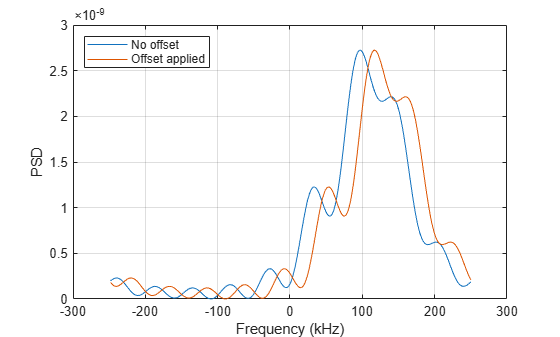Generate output samples and matched filter coefficients of a linear FM pulse waveform at a 50 kHz frequency offset.

waveform = phased.LinearFMWaveform('SweepBandwidth',1e5, ... 'PulseWidth',5e-5,'OutputFormat','Pulses', ... 'FrequencyOffset',5e4,'CoefficientsOutputPort',true); [wav,coeff] = waveform();

Create a matched filter that applies the coefficients as an input argument. Use the coefficients when applying the matched filter to the waveform. Plot the waveform and matched filter outputs.

mf = phased.MatchedFilter('CoefficientsSource','Input port'); mfOut = mf(wav,coeff); subplot(211),plot(real(wav)); xlabel('Samples'),ylabel('Amplitude'),title('Waveform Output'); subplot(212),plot(abs(mfOut)); xlabel('Samples'),ylabel('Amplitude'),title('Matched Filter Output');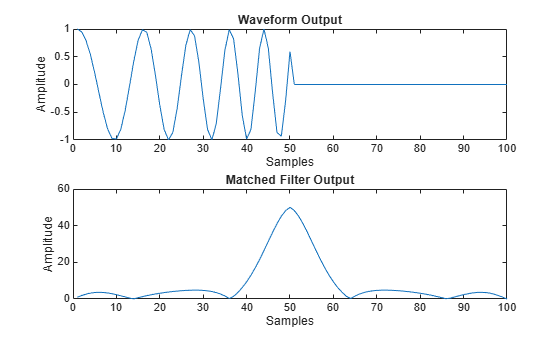Determine the bandwidth of a linear FM pulse waveform. The default value for an LFM waveform is 100 kHz.

waveform = phased.LinearFMWaveform; bw = bandwidth(waveform)
bw = 100000 

 Levanon, N. and E. Mozeson. Radar Signals. Hoboken, NJ: John Wiley & Sons, 2004.

 Richards, M. A. Fundamentals of Radar Signal Processing. New York: McGraw-Hill, 2005.